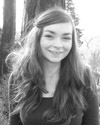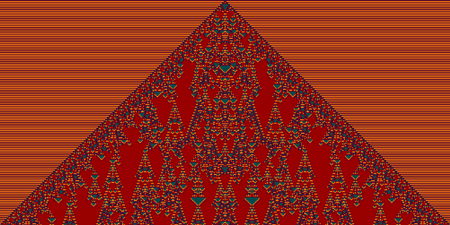#Wolfram Summer School

# Alumni# Isabella Thiesen

Summer School

Class of 2011

## Bio

Isabella studies mathematics at Freie Universitaet Berlin, Germany and will graduate this summer. She just handed in her diploma thesis on overlap circle packings and the discrete Ricci flow and is now looking for new challenges.

She is interested in discrete differential geometry and is looking forward to applying NKS to this field. Isabella likes colors, patterns, water, languages, traveling, and discovering something new.

## Project: Networks as Manifolds

This project is related to the section “Space as a Network” in Chapter 9 of the NKS book.

We start with very simple networks consisting only of a couple of nodes and evolve these according to specified rules. Although these networks are given purely in terms of combinatorics, we can think of imposing a distance measure by, e.g., taking the distance between any two nodes as the shortest number of edges connecting the two. We can then use this measure to consider linear plots of the network graphs, where we start at one node and plot the graph in ascending distance order of the nodes (see p. 479). The function describing the resulting pattern might then contain information about the structure underlying the network, which could relate to some well-known manifold.

For example, in the case of a network representing Euclidean n-space, the number of nodes out to distance r from a node should be O(r^n), such as the volume function behaves in the continuous case for n-dimensional balls. As done in figure(f) on p.479, we can interpret graph functions that do not mimic this behavior as curved. For common 2-dimensional manifolds, such as the torus or bretzel model, we want to investigate the behaviour of its graph functions for different refinements of their cell complexes.

The focus of this work lies on the identification of 2-manifolds underlying a given selection of evolving networks. We want to make use of the graph function and think of other techniques to find out which manifolds can be produced by simple replacement rules. The goal is to be able to identify the manifolds for the networks that come from network substitution systems.

You may view the results of this project as a PDF.

## Favorite Four-Color Totalistic Cellular Automaton

Rule 604000• 22nd Annual Wolfram Summer School
• Bentley University, Waltham, MA, USA
• June 23–July 11, 2024